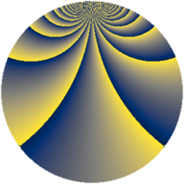# Properties

 Label 1815.2.wLevel $1815$ Weight $2$ Character orbit 1815.w Rep. character $\chi_{1815}(323,\cdot)$ Character field $\Q(\zeta_{20})$ Dimension $1600$ Sturm bound $528$

# Related objects

## Defining parameters

 Level: $$N$$ $$=$$ $$1815 = 3 \cdot 5 \cdot 11^{2}$$ Weight: $$k$$ $$=$$ $$2$$ Character orbit: $$[\chi]$$ $$=$$ 1815.w (of order $$20$$ and degree $$8$$) Character conductor: $$\operatorname{cond}(\chi)$$ $$=$$ $$165$$ Character field: $$\Q(\zeta_{20})$$ Sturm bound: $$528$$

## Dimensions

The following table gives the dimensions of various subspaces of $$M_{2}(1815, [\chi])$$.

Total New Old
Modular forms 2304 1856 448
Cusp forms 1920 1600 320
Eisenstein series 384 256 128

## Trace form

 $$1600q + 10q^{3} + 12q^{6} + 20q^{7} + O(q^{10})$$ $$1600q + 10q^{3} + 12q^{6} + 20q^{7} + 40q^{10} + 20q^{12} + 4q^{13} + 22q^{15} + 328q^{16} - 2q^{18} + 24q^{21} + 60q^{25} + 22q^{27} - 8q^{28} + 26q^{30} + 8q^{31} + 124q^{36} - 28q^{37} - 52q^{40} - 14q^{42} - 52q^{45} - 14q^{48} + 116q^{51} - 12q^{52} - 30q^{57} - 136q^{58} - 98q^{60} + 14q^{63} - 400q^{67} - 56q^{70} - 92q^{72} + 80q^{73} + 38q^{75} + 176q^{76} - 100q^{78} - 72q^{81} - 28q^{82} + 12q^{85} + 36q^{87} - 16q^{90} - 128q^{91} + 26q^{93} - 152q^{96} + 108q^{97} + O(q^{100})$$

## Decomposition of $$S_{2}^{\mathrm{new}}(1815, [\chi])$$ into newform subspaces

The newforms in this space have not yet been added to the LMFDB.

## Decomposition of $$S_{2}^{\mathrm{old}}(1815, [\chi])$$ into lower level spaces

$$S_{2}^{\mathrm{old}}(1815, [\chi]) \cong$$ $$S_{2}^{\mathrm{new}}(165, [\chi])$$$$^{\oplus 2}$$